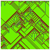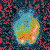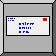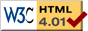# Due March 25, 2019

## General Description

• In this project you will be investigating the associative memory capacity of a Hopfield network.  You will use N = 100 neurons with no self-coupling.
• For additional information, see Bar-Yam Section 2.2.6 (pp. 312–19, available online).
• See Dr. Van Hornweder’s web page <web.eecs.utk.edu/~mclennan/Classes/420-527/projects/project3/hopfieldnet.html> for additional information, hints, tips, etc.
• You are expected to implement the software to do these experiments; turn it in with your report.

## For COSC 420 Credit

• Perform a series of experiments as follows:
1. Generate 50 random bipolar vectors (N = 100).
2. For p = 1, ..., 50, imprint the first p patterns on a Hopfield net.  (The rule for imprinting p patterns is given on slide 63 in Part 3A.)
3. Determine pstable, the number of stable imprinted patterns.  An imprinted pattern is considered unstable if any of its bits are unstable, that is, if bit i is of opposite sign to its local field hi.  Therefore, after imprinting the first p patterns, test each imprinted pattern k (k = 1, …, p) as follows: Initialize the cells to pattern k and compute the local fields hi of each bit. Compare each bit with local field to test for stability.  Repeat for each of the p patterns.   (The computation of the local field is described on slides 5–6 in Part 3A.)
4. Compute the probability of an imprinted pattern being stable, Pstable = pstable / p.  (Note that p, Pstable, and pstable are different; this is Bar-Yam’s notation, which I’ve retained for consistency with his book.)
5. Repeat steps 2–4 for p = 1, ..., 50 and keep track of Pstable for each value of p.
6. Repeat the forgoing for several sets of 50 random patterns and average over them.
• As a result of your simulations, you should generate two graphs:  First, a plot of the fraction of unstable imprints as a function of the number of imprints (p = 1, ..., 50).  Second, a plot of the number of stable imprints as a function of the number of imprints (same range).
• For examples, see Bar-Yam Section 2.2.6 (pages 312–14); your program should be able to generate graphs comparable to Figs. 2.2.5 and 2.2.6 on p. 315 (but it is not necessary to normalize to p).
• You should hand in (1) your program, (2) your graphs or other output, and (3) a discussion of your results and their implications.
• For extra credit, do some or all of the activities described below For COSC 427 and 527 Credit.

## For COSC 427 and 527 Credit

• You should do everything expected For COSC 420 Credit (above).
• In addition, you should investigate the size of the basins of attraction as a function of the number of imprinted patterns (Bar-Yam, Section 2.2.6, pp. 314–16).  In other words, you should generate graphs similar to Fig. 2.2.7 (p. 315).  Here is the procedure:<note>

1. Generate 50 random patterns.
2. For p = 1, …, 50, imprint the first p test patterns, as for your stability tests.
3. For each of the p imprinted patterns, estimate the size of its basin of attraction, as follows.
4. If the pattern is unstable, then the size of its basin is 0.
5. Otherwise, you will estimate how many bits you can change in the imprinted pattern and still have it converge to the correct stable state in a certain fixed number of steps (n = 10).
6. More specifically, to estimate the size of the basin of imprinted pattern k (k = 1, …, p), generate a random permutation of the numbers 1, …, 100; let Li be the i-th number in this permutation.  This list gives you the order in which you will change the bits of pattern k.  You will change at most 50 bits, since that is the maximum size of a basin (Why?).  Therefore, all you need is L1 to L50.
7. For the i-th iteration, you will flip bits L1 , L2, …, Li of pattern k, initialize the net to the resulting modified pattern, and then see if the net converges to pattern k within 10 cycles (that is, 10 updates of all the cells).
8. Estimate the size of the basin to be the minimum i for which the modified pattern doesn’t converge to pattern k.
9. Repeat steps 5–7 for several different random permutations of 1, …, 100, where Li is the i-th number in this permutation.  Average the results to estimate the basin size for pattern k.
10. Repeat steps 3–9 for each imprinted pattern k.   Compute a basin histogram, which counts the number of imprinted patterns with each different basin size 0, 1, …, 50.
11. Repeat steps 3–10 for p = 1, …, 50.  Plot the basin histogram for each value of p.  (You can put them all on one graph, as in Bar-Yam Fig. 2.2.7, if you like.)

• Hand in your programs, graphs, and a discussion of your results. Please follow the report template that we have provided on Canvas, including an introduction and brief methodology/theory sections (with relevant equations).
• For extra credit, you can investigate the effect of noise (pseudo-temperature) on retrieval of imprinted patterns (Bar-Yam, Question 2.2.2, pp. 316–19).  In other words, you should generate graphs comparable to Fig. 2.2.8 (p. 318).  Use the stochastic update rule we discussed in class (recall β = 1/T):
Pr{si' = +1} = σ(hi),
Pr{si' = –1} = 1 – σ(hi),
where σ(h) = 1 / [1 + exp(–2h / T)].
• <note> The procedure for  computing the distribution of basin sizes is given on pp. 314–15 of Bar-Yam, but I don’t  recommend that you look at it, because the notation is not very good.  However, if you do look at it, please note that there is an error in the first line of Eq.  2.2.39.  The  i subscript (on ξμi or xi^mu_i) should be  j (that is, ξμj or xi^mu_j).

If you have any other questions, please post them on Piazza.Return to CS 420/427/527 home pageReturn to MacLennan’s home pageSend mail to Bruce MacLennan / MacLennan@utk.eduThis page is web.eecs.utk.edu/~mclennan/Classes/420-527/projects/project3/Project-3.html
Last updated: 2019-03-02.